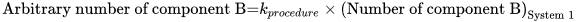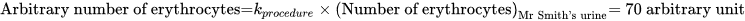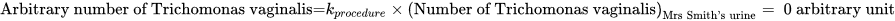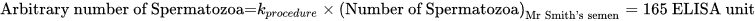Select Page

# Arbitrary number (arb.num.)

Arb.num.

### Definition in words

1. Arbitrary number of component B in system 1 (Ratio scale, see Definition by equation).
2. Arbitrary number of component B in system 1 compared with arbitrary number of component B in reference system (Ordinal scale).

Note 1: This concept has two definitions.

Note 2: The reference system is defined by the laboratory.

### Definition by equation

1.Note: kprocedure is a constant defined by the measurement procedure (there is an approximately proportionality to a kind-of-quantity from the International System of Quantities)

### Result type

Ordinal scale
• Value set is ranked accoding to magnitude
• The value set and the limit of the value set are defined by the laboratory
• The value set can be either numerical values or words.Example 1Value set={none < some < high < very high}Example 2Value set={low < normal< high }

Example 3

Value set={0 < 1 < 2}

Example 4

Value set={negative < positive}

Example 5

Value set={+ < ++ < +++}

• Algebraic operations are not allowed (Ref. 2, 1.26)
• Possible comparison of values (a<b, a=b or a>b)
Rational scale
• Value sets are positive numerical values
• Numerical values from 0-∞
• Algebraic operations are allowed
• Possible comparison of values (a=nb; a≠nb)

### Unit

International unit (IU) or procedure defined unit (p.d.u.) (non-SI units)

### Special rule(s) in the NPU syntax

“Procedure” is stated in the specification to the kind-of-property in the NPU definition.

“Procedure” indicates that the measurement procedure must be stated somewhere else than the NPU code and there may be difficulties in comparing values for the same NPU code.

If the ratio scale is attributed to the NPU definition, the unit can be non-SI unit.

If the unit is an International Unit (IU), an international certified reference material (e.g. WHO reference material) is specified in the specification to the kind-of-property.

If there exist no international certified reference material, the laboratory can specify the unit at the placeholder, procedure defined unit.

If ordinal scale is attributed, no unit is stated.

### Reference

1. Ferard G, Dybkaer R, Fuentes-Arderiu X. Compendium of Terminology and Nomenclature of Properties in Clinical Laboratory Sciences : Recommendations 2016. 1 ed: Royal Society of Chemistry; 2016. 182 p.
2. Mesures BIdPe. International Vocabulary of Metrology – Basic and General Concepts and Associated Terms VIM 3rd edition JCGM 200:2012 (JCGM 200:2008 with minor corrections). https://www.bipm.org: Bureau International des Poids et Mesures; 2008.

### Allegology

Example 1 (ordinal)
Generic abbreviated form NPU02480 Ercs(B)—Immunoglobulin E; arb.num.(proc.) = ?
Full form with a result NPU02480 Erythrocytes(Blood)—Immunoglobulin E; arbitrary number(procedure) = high
Abbreviated form with a result NPU02480 Ercs(B)—Immunoglobulin E; arb.num.(proc.) = high
Written expression The arbitrary number of Immunoglobulin E of Erythrocytes in Mr Smith’s blood is [high] compared with the arbitrary number of Immunoglobulin E of Erythrocytes in a reference system. The measurement is performed according to a procedure defined by the given laboratory.
Notes
1. The reference system is defined by the laboratory.
2. “High” is a value according to a value set defined by the laboratory.

### Clinical Chemistry

Example 2 (ratio)
Generic abbreviated form NPU18636 U—Ercs; arb.num.(proc.) = ? (p.d.u.)
Full form with a result NPU18636 Urine—Erythrocytes; arbitrary number(procedure) = 70 arbitrary unit
Abbreviated form with a result NPU18636 U—Ercs; arb.num.(proc.) = 70 arbitrary unit
Written expression The arbitrary number of Erythrocytes in Mr Smith’s urine is  arbitrary unit. The measurement is performed according to a procedure defined by the given laboratory.
EquationNotes “Arbitrary unit” was chosen by a local laboratory as a proprietary measurement unit and not an accepted international unit
Example 3 (ordinal)
Generic abbreviated form NPU04063 Lkcs(B)—Aldosterone receptor; arb.num.(proc.) = ?
Full form with a result NPU04063 Leukocytes(Blood)—Aldosterone receptor; arbitrary numbers(procedure) = 2
Abbreviated form with a result NPU04063 Lkcs(B)—Aldosterone receptor; arb.num.(proc.) = 2
Written expression The arbitrary number of Aldosterone receptor of Leukocytes in Mr Smith’s blood is  compared with the arbitrary number of Aldosterone receptor of Leukocytes in a reference system. The measurement is performed according to a procedure defined by the given laboratory.
Notes
1. The reference system is defined by the laboratory.
2. “2” is a value according to a value set defined by the laboratory.

### Clinical Immunology and Blood Bank

Example 4 (ordinal)
Generic abbreviated form NPU20206 Ercs(B)—Erythrocyte BARC antigen; arb.num.(proc.) = ?
Full form with a result NPU20206 Erythrocytes(Blood)—Erythrocyte BARC antigen; arbitrary number(procedure) = high
Abbreviated form with a result NPU20206 Ercs(B)—Erythrocyte BARC antigen; arb.num.(proc.) = high
Written expression The arbitrary number of Erythrocyte BARC antigen of Erythrocytes in Mr Smith’s blood is [high] compared with the arbitrary number of Erythrocyte BARC antigen of Erythrocytes in a reference system. The measurement is performed according to a procedure defined by the given laboratory.
Notes
1. The reference system is defined by the laboratory.
2. “High” is a value according to a value set defined by the laboratory.

### Clinical Microbiology

Example 5 (ratio)
Generic abbreviated form NPU18642 U—Trichomonas vaginalis; arb.num.(proc.) = ? (p.d.u.)
Full form with a result NPU18642 Urine—Trichomonas vaginalis; arbitrary number(procedure) = 0 arbitrary unit
Abbreviated form with a result NPU18642 U—Trichomonas vaginalis; arb.num.(proc.) = 0 arbitrary unit
Written expression The arbitrary number of Trichomonas vaginalis in Mrs Smith’s urine is  arbitrary unit.The measurement is performed according to a procedure defined by the given laboratory.
EquationNotes “Arbitrary unit” was chosen by a local laboratory as a proprietary measurement unit.and not an accepted international unit.

### Reproduction and Fertility

Example 6 (ratio)
Generic abbreviated form NPU18891 Sem—Spermatozoa; arb.num.(proc.) = 165 (p.d.u.)
Full form with a result NPU18891 Semen—Spermatozoa; arbitrary number(procedure) = 165 ELISA unit
Abbreviated form with a result NPU18891 Sem—Spermatozoa; arb.num.(proc.) = 165 Elisa unit
Written expression The arbitrary number of Spermatozoa in Mrs Smith’s semen is  ELISA unit. The measurement is performed according to a procedure defined by the given laboratory.
EquationNotes “ELISA unit” was chosen by a local laboratory as a proprietary measurement unit.and not an accepted international unit.

### Reference

NPU database, https://www.ifcc.org/ifcc-scientific-division/sd-committees/c-npu/npusearch/ and http://www.labterm.dk/default.aspx

• # Kind-of-property

• ## Kind-of-quantity

### Reference

• Dybkaer R. Concept system on ‘quantity’: formation and terminology. Accredit Qual Assur. 2013;18(3):253-60.
• Dybkaer R. ISO terminological analysis of the VIM3 concepts ‘quantity’ and ‘kind-of-quantity’. Metrologia. 2010;47(3):127-34.
Date Term Note
1996-01-01 Arbitrary number Term established

QU50151